#### 2001 (Volume 14)Number 1Number 2Number 3Number 4

#### 2000 (Volume 13)Number 1Number 2Number 3Number 4

#### 1999 (Volume 12)Number 1Number 2Number 3Number 4

#### 1998 (Volume 11)Number 1Number 2Number 3Number 4

#### 1997 (Volume 10)Number 1Number 2Number 3Number 4Number 5

#### 1996 (Volume 9)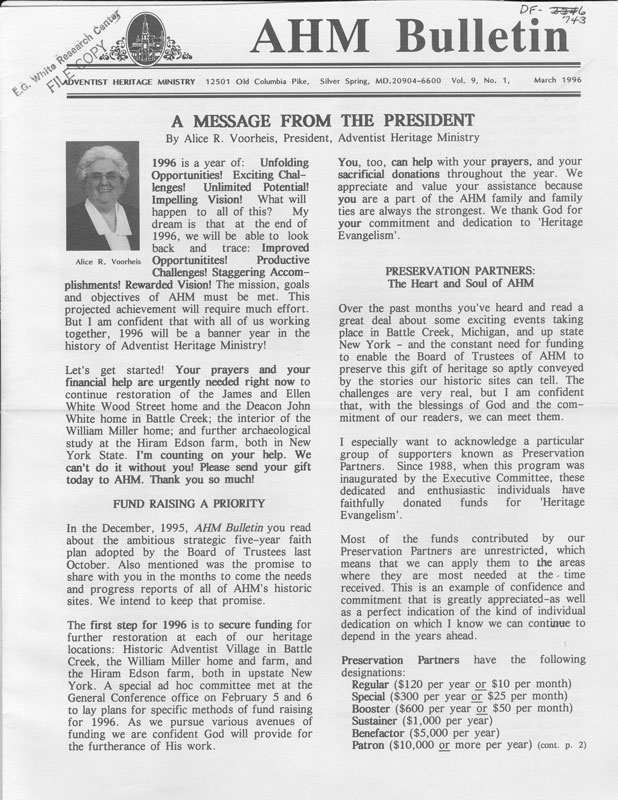Number 1Number 2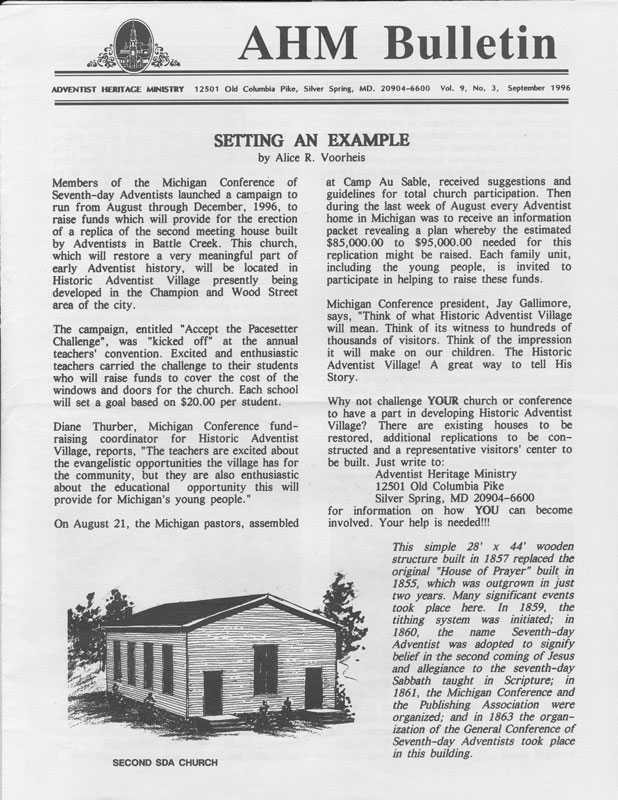Number 3Number 4

#### 1995 (Volume 8)Number 1Number 2Number 3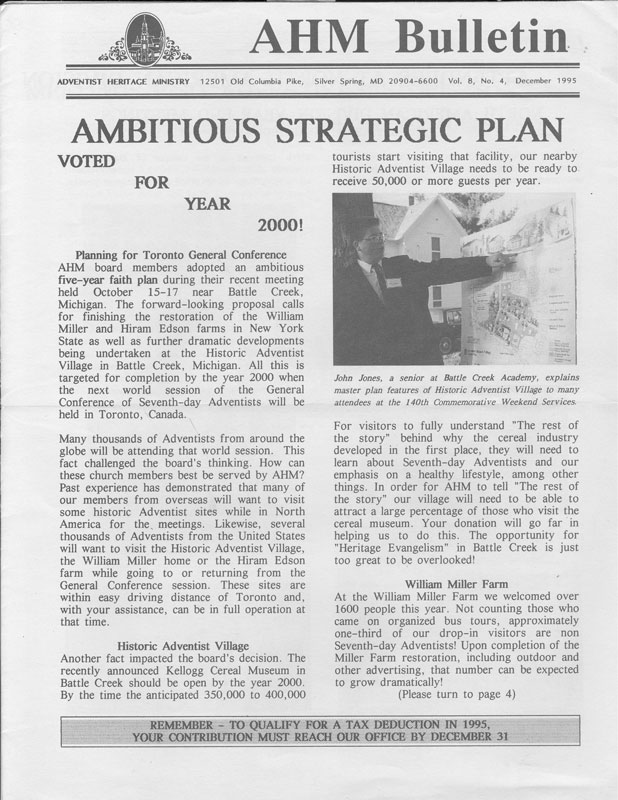Number 4Number 5

#### 1994 (Volume 7)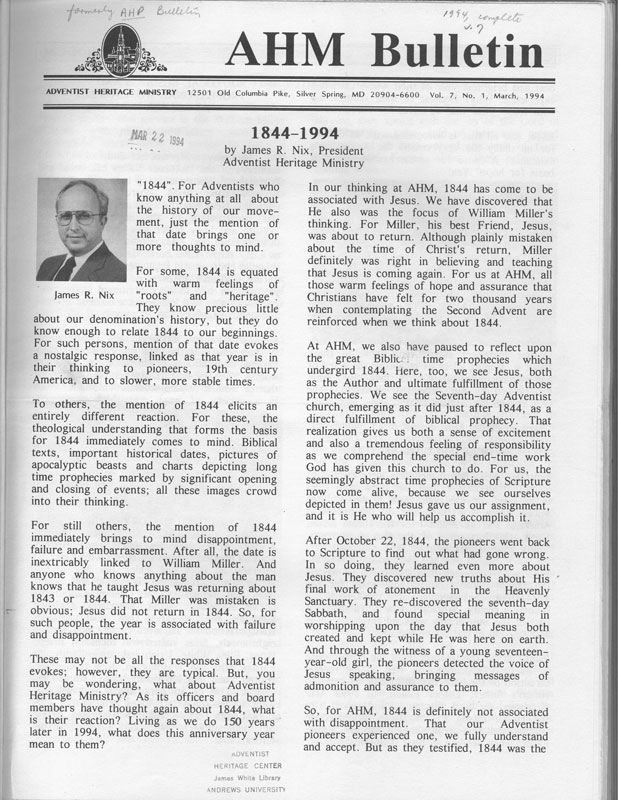Number 1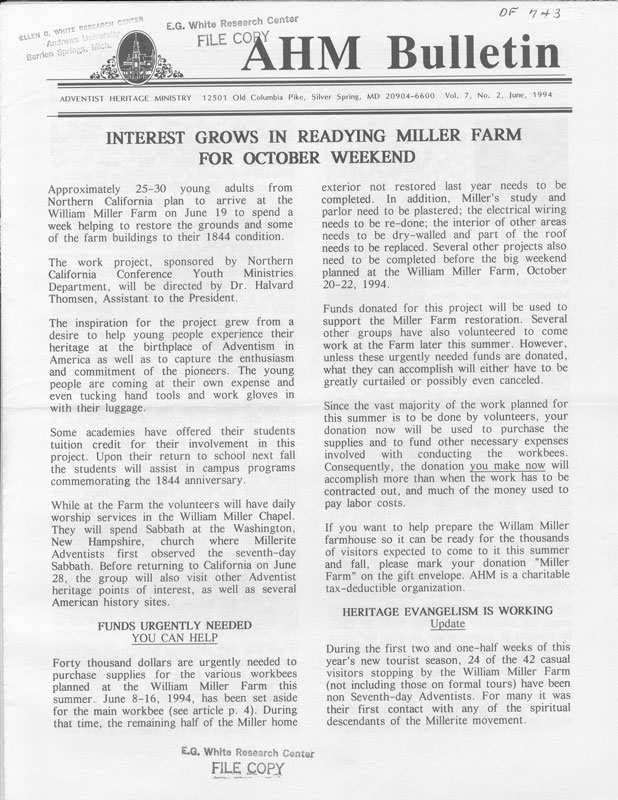Number 2Number 3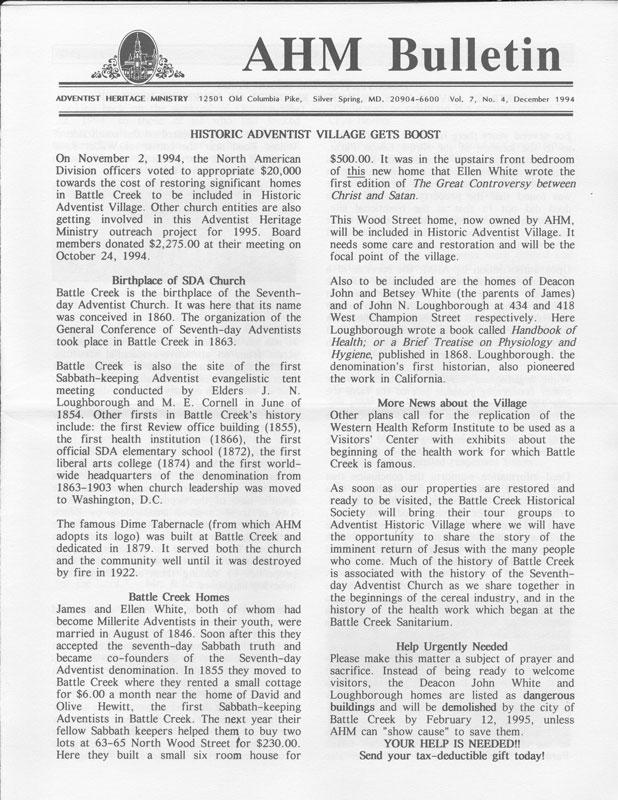Number 4Number 5

#### 1993 (Volume 6)Number 1Number 2Number 3

#### 1992 (Volume 5)Number 1Number 2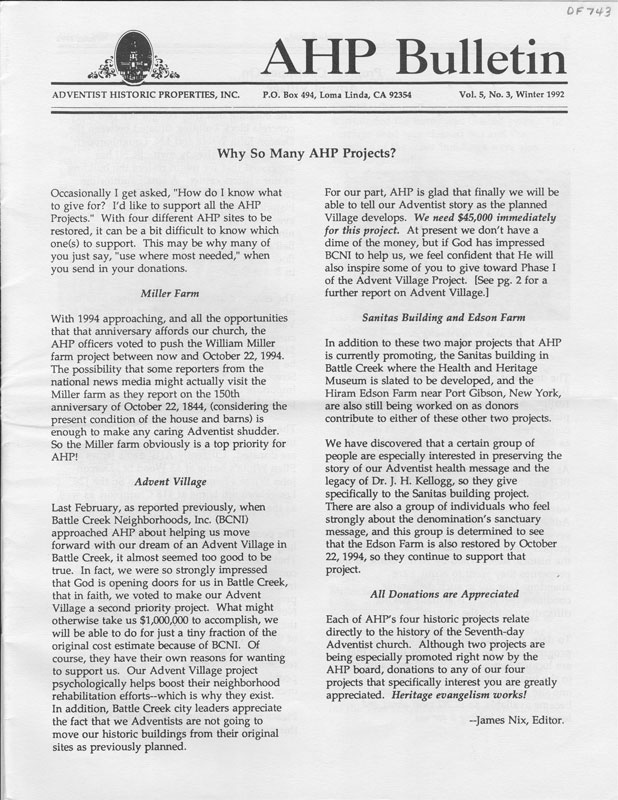Number 3